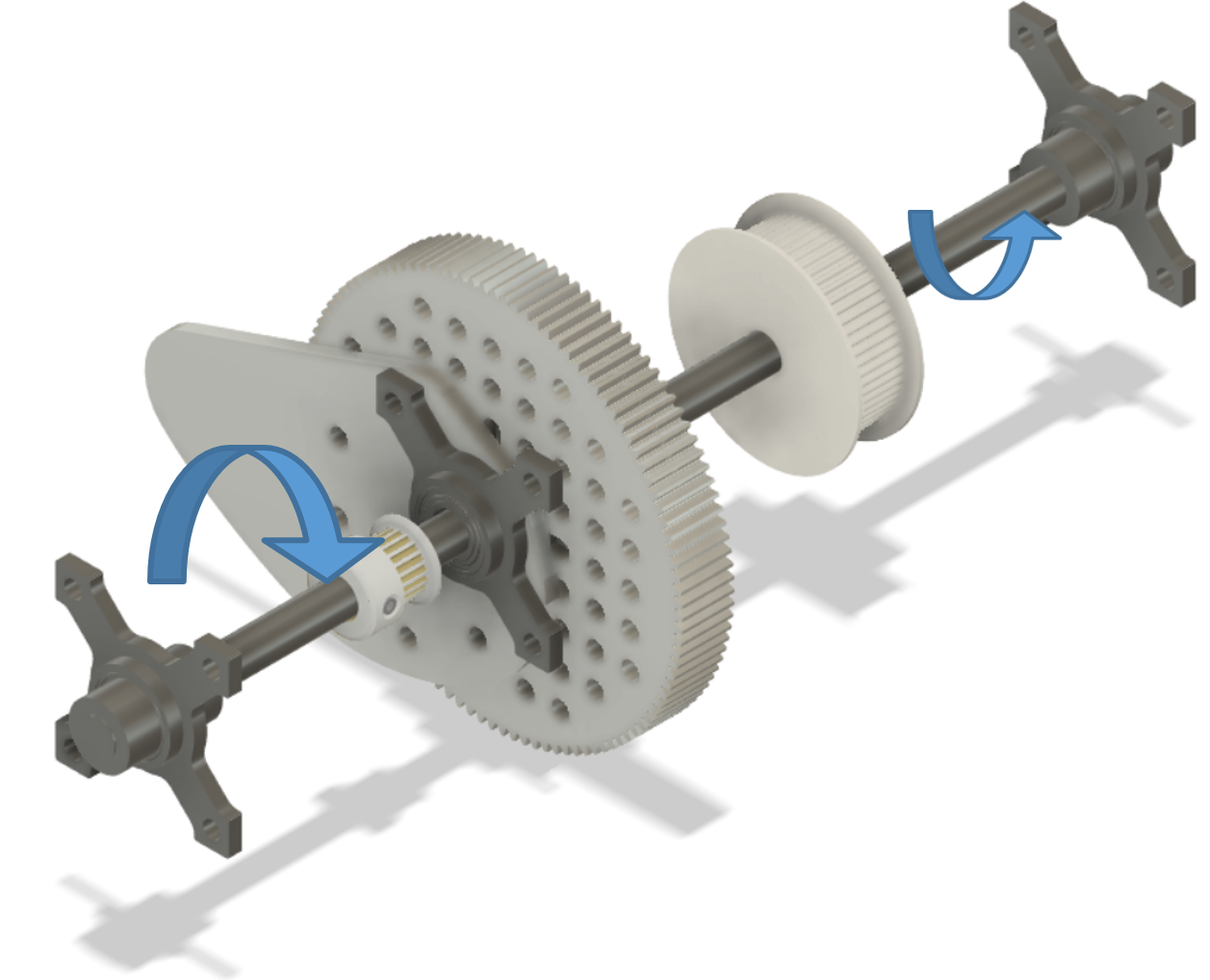# Calculating Torque at the Elbow

Tags: think and design
Personhours: 4
Calculating Torque at the Elbow By Bhanaviya

### Task: Design an equation to model the torque at the elbow linearlyIn order to maximize our robot performance, we need to be able to use motors and gears with the most ideal gear ratios. This means having the right amount of torque to produce the most efficient performance out of our robot arm. As the arm extends, there is quite a bit of torque on the elbow. We want to model this torque as a function which will allow us to better analyze how much rotational force is being exerted on the elbow and whether the gear ratio of the gears at the elbow could be improved by using a different gear set.

The torque at the elbow is a dynamic variable that changes as the arm is extended further and further. As such, we decided to model this equation as a function. Torque is generally T = Frsin(theta). F is force which can be derived by multiplying the mass of the arm (m) with g, the acceleration due to gravity. g can be otherwise defined as g = G, the universal gravitational constant, * (m/r * r). r is the distance from the center of mass of the arm when fully retracted to the axis of rotation, which is, in this case, the elbow. This value can be defined by 170.4 meters. However, since the center of mass changes as the arm extends, r is not a constant and as such can be modeled as C + x, where C is the constant for the center of mass when the arm is fully retracted increasing by x, wherein x is the value in meters by which the arm extends. C can be defined by 2358.68 grams. As the arm is extended, the axis of rotation is in motion so theta, the angle in degrees between the vertical and the position of the arm changes linearly and as such, cannot be represented as a constant. However, since this angle is more difficult to derive, and since the angle between the position of the arm and the horizontal is already shown in the code for the controls operating the elbow, theta can simply be stated as 90 - theta_0, wherein theta_0 is the angle between the horizontal and the position of the arm. The torque due to gravity at the elbow can be represented as the function T = 2358.68 grams g(170.4 meters + x)sin(90 - theta_0) wherein theta_0 and x are parameters which can be taken from the articluation positions.

### Next Steps

Being able to model torque as a function will allow us to understand how much torque is needed for the robot to stack a certain number of stones. By identifying the non-constant values of the torque function, we will be able to analyze what specific values produce the best robot performance, as well as whether that performance can be improved by lightening the load on the arm.

Date | January 1, 2020